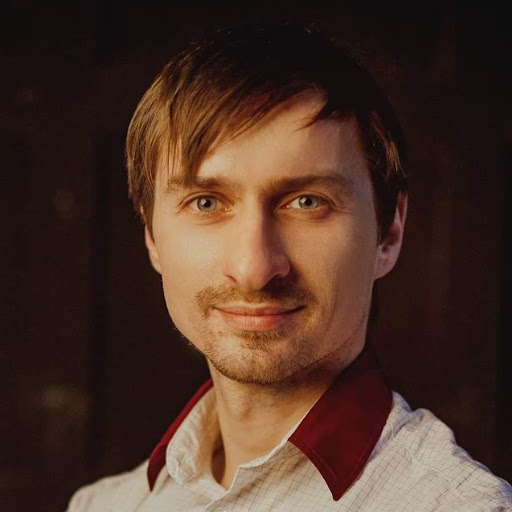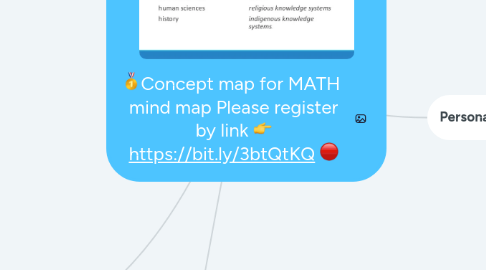# 🥇Concept map for MATH mind map Please register by link 👉 https://bit.ly/3btQtKQ 🔴Oleg Gavryliuk

Начать. Это бесплатно
или регистрация c помощью Вашего email-адреса🥇Concept map for MATH mind map Please register by link 👉 https://bit.ly/3btQtKQ 🔴 создатель## 1. Scope & Application (jam)

### 1.1. Three views

1.1.1. Plativist

1.1.1.1. Seen as code to understand the world around us

1.1.2. Constructivists

1.1.2.1. Mathematics, in its.nature, is abstract and artificial

1.1.3. Formalist

1.1.3.1. An abstract game based on made-up rules

### 1.2. Can Math predict the futre?

1.2.1. In certain ways, calculus can be used to see predicted change

1.2.2. Euler's number can predict growth

1.2.3. Benford's law, states that numbers beginning with 1 are much more prevalent

1.2.3.1. The point is there are always patterns to analyze

1.2.3.1.1. This means that if we find the pattern, we can predict some things that happen in the future

### 1.4. Computer algorithms

1.4.1. e.g Monte Carlo Method

1.4.2. Ethical Issues

1.4.2.1. If person A uses Person B's code to solve a problem, who gets the credit?

## 2. Methodology [jam]

### 2.1. Law of excluded middle

2.1.1. Everything is true or false

### 2.2. Logical Deduction

2.2.1. tautologies

2.2.1.1. Answer which is correct in all circumstances

### 2.3. Proofs

2.3.1. Prove beyond doubt

2.3.1.1. Evidence is not good enough, must be undebatable

2.3.2. Types of proofs

2.3.2.1.1. Stating that the opposite is false and therefore the former is true

2.3.2.2. Substitution (Direct)

2.3.2.2.1. Placing an example into a situation

2.3.2.3. Mathematical induction principle

2.3.2.3.1. P(n) is true, then P(n+1) is true

2.3.2.4. Pigeon hole principle

2.3.2.4.1. If you have more pigeons than pigeon holes, there will be pigeon holes with more than one pigeon

### 2.4. Axioms

2.4.1. Mathematical theory

2.4.1.1. Generally accepted amongst mathematicians

2.4.1.1.1. Theorems are made from this

2.4.2. Of the set theory

2.4.2.1. Generally accepted amongst mathematicians

2.4.3. Previous truths, and initial statements

### 2.5. Research

2.5.1. Blue skies research

2.5.1.1. Research for the sake of researching, no clear goal

2.5.2. Relating different areas of research

2.5.2.1. Filling in gaps

2.5.3. Attacking a famous problem

2.5.3.1. Looking at the existing approaches and approaching a problem in a different way

2.5.4. Applying a standard method to a standard type of problem

2.5.4.1. Simplifying the problem and ultimately trying to get outcomes to apply to future research

## 3. Personal Knowledge

### 3.1. What is the nature of the contribution of individuals you know personally to this area, in terms of your experience?

3.1.1. The contributions are generally quite respected within the mathematical community, and is usually taken as truth. Generally the people who contribute also leads to people expanding more on their contributions, and thus many contributions within math are based on other people's contributions.

### 3.2. What responsibilities rest upon YOU by virtue of YOUR knowledge in this area?

3.2.1. It is my responsibility to make sure that when people are exposed to the area of math, that they are not forced into using one way of solving the issue. There are many ways to solve the equation 2+2, for example. 2 can be written as 1+1+1+1 and it can also be 2*2. The point is that these different ways of solving a problem can help a person grasp a better understanding of math and help them to respect it more, as they are not bound to this idea that math is boring and has too many rules and regulations for any sort of imagination.

### 3.3. What are the implications of this area of knowledge in terms of YOUR individual perspective?

3.3.1. Math is still surprisingly subjective. Some problems have different ways of solving them, which can sometimes lead to different answers. Because of this, certain answers may be valued more over others in order to support their ideas. Math can also be a way of exploring imagination and different perspectives. A lot of the theories made in Math were created because of peoples' imaginations, which lead to the making of assumptions to then create their own theorem.

### 3.4. What assumptions underlie YOUR own approach to this knowledge?

3.4.1. In mathematics, there is a growing assumption that there is always one answer to a question. Because of this, a lot of the knowledge about math can be limited when it comes to approaching different problems. People are taught to solve certain problems in certain ways and we are not given the freedom of looking at a problem from a different perspective, which might end you up with a different answer. Because of this, my approach to Mathematics can be considered quite binary, as I would approach questions with the assumption that there is only one right answer.

### 3.5. Consider the WOKs in relation to your experiences, how have these affected what and how you know in this AOK?

3.5.1. I definitely make use of Intuition, Imagination and Faith the most. Intuition helps me to solve a lot of math problems. Imagination allows me to think outside the box, especially with problems that can't be linked to something in real life, like algebra and such. I also have a lot of faith that all the mathematicians contributions are true and am less likely to question them.

## 4. Language and Concepts (jam

### 4.1. Is mathematics a universal language?

4.1.1. Mathematics is the study of universal truths, but does that mean the language used is universal?

4.1.1.1. Arecibo message

### 4.2. Set theory

4.2.1. How does it impact language in maths

4.2.2. How does it affect produced knowledge

### 4.3. Infinities and continuum hypothesis

4.3.1. Aleph-nought

4.3.1.1. Countable infinity of integers

4.3.2. Aleph-one

4.3.2.1. Uncountable infinity of integers

### 4.4. Metaphors and symbols

4.4.1. Different ways of writing one

4.4.1.1. Can be based off how accurate you want data to be

4.4.1.2. Ways of providing a simpler explanation to an issue

4.4.1.3. Can help to approach a problem differently

### 4.5. Schema

4.5.1. Mental structures used in mathematics

### 4.6. Linear notation and higher dimensional algebra

4.6.1. Symbols are related not only from left and right

4.6.1.1. Also related from up and down

4.6.1.1.1. Even outside of the page

4.6.2. Can help in modelling geometric situations

4.6.3. New proofs, methods, and theorems can be formulated

## 5. Historical Development (Jam)

5.1.1. Theorems are made to explain said conjectures

5.1.1.1. Compared to NS, this is more inductive rather than deductive

5.1.2. Or Paradigm Shifts happen and conjectures are disproven

### 5.2. Key points in mathematical history

5.2.1. The invention of zero

5.2.2. Axiomization

5.2.3. Fibonacci and his work

5.2.4. The invention of algebra

5.2.5. Analytical geometry

5.2.6. Fermat's last theorem

5.2.7. Pascal's triangle

5.2.8. Counting in binary and computers

5.2.9. Babbage difference engine

5.3.1. For example Cantor's set theory

## 6. Concept map for Mathematics Mind Map

### 6.14. 3.1. line graphs

6.14.1. Math Mind Map